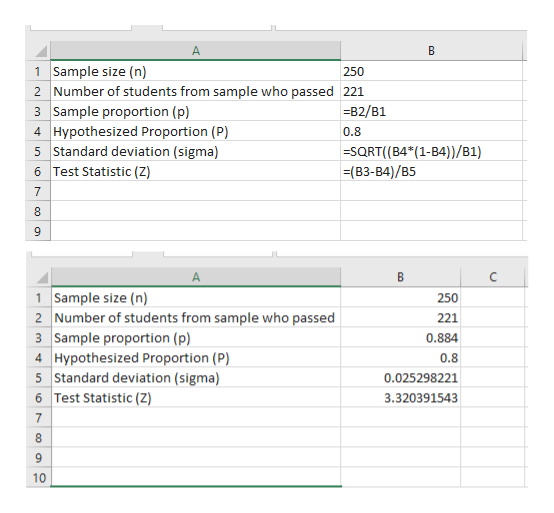# A college professor claims that the proportion of students passing a statistics course is 80%. To test this claim, a random sample of 250 students who previously took the course is taken and it is determined that 221 students passed the course.The following is the setup for this hypothesis test:H0:p = 0.80Ha:p ≠ 0.80Find the test statistic for this hypothesis test for a proportion and round your answer to 2 decimal places.Provide your answer below:TEST STATISTIC=

Question
166 views

A college professor claims that the proportion of students passing a statistics course is 80%. To test this claim, a random sample of 250 students who previously took the course is taken and it is determined that 221 students passed the course.

The following is the setup for this hypothesis test:

H0:p = 0.80

Ha:p ≠ 0.80

Find the test statistic for this hypothesis test for a proportion and round your answer to 2 decimal places.

TEST STATISTIC=

check_circle

Step 1

Test statistic for Z test can be calculated using the formula shown below.

Step 2

Calculations can be performed on...help_outlineImage TranscriptioncloseA 1 Sample size (n) 250 2 Number of students from sample who passed 221 3 Sample proportion (p) B2/B1 4 Hypothesized Proportion (P) 0.8 5 Standard deviation (sigma) -SQRT((B4*(1-B4))/B1) (B3-B4)/B5 6 Test Statistic (Z) 7 8 1 Sample size (n) 2 Number of students from sample who passed 3 Sample proportion (p) 4 Hypothesized Proportion (P) 5 Standard deviation (sigma) 6 Test Statistic (Z) 250 221 0.884 0.8 0.025298221 3.320391543 7 8 10 co co a fullscreen

### Want to see the full answer?

See Solution

#### Want to see this answer and more?

Solutions are written by subject experts who are available 24/7. Questions are typically answered within 1 hour.*

See Solution
*Response times may vary by subject and question.
Tagged in

### Hypothesis Testing